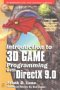# Maths - Vector Subspaces

For 'H' to be a subspace of a vector space 'V' it must have 3 properties:

• The zero vector of V is in H.
• For each 'u' and 'v' in H then v+u is also in H (closed under +).
• For each 'v' in H and scalar 's' then s*v is also in H (closed under scalar multipication).

### Example of a Subspace

V
 x y z
a vector space in 3 dimensions x,y and z
H
 x y 0
a subspace of V formed by setting the z dimenstion to zero.

### Theorm

If v1…vp are in a vector space V, then span{v1…vp}is a subspace of V.

### Lattice of Vector Subspaces

We can represent the relationships between a whole set of subspaces by a lattice structure (lattices are described on this page).One way to encode this lattice structure of algebras is, as a Clifford algebra, as explained on this page.

 metadata block see also: Correspondence about this page Book Shop - Further reading. Where I can, I have put links to Amazon for books that are relevant to the subject, click on the appropriate country flag to get more details of the book or to buy it from them.Introduction to 3D Game Programming with DirectX 9.0 - This is quite a small book but it has good concise information with subjects like, maths introduction and picking.If you are interested in 3D games, this looks like a good book to have on the shelf. If, like me, you want to have know the theory and how it is derived then there is a lot for you here. Including - Graphics pipeline, scenegraph, picking, collision detection, bezier curves, surfaces, key frame animation, level of detail, terrain, quadtrees & octtrees, special effects, numerical methods. Includes CDROM with code. This book contains more mathematically rigorous methods for picking than described above. Other Math Books Specific to this page here:

This site may have errors. Don't use for critical systems.